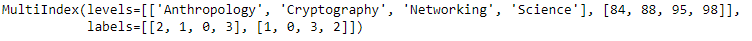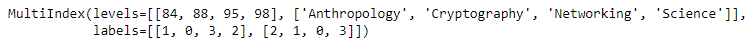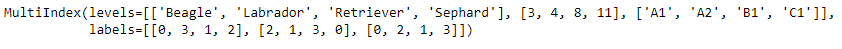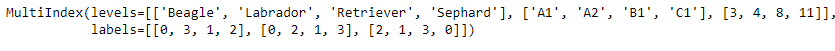GeeksforGeeks App
Open AppBrowser
Continue

# Python | Pandas MultiIndex.reorder_levels()

Python is a great language for doing data analysis, primarily because of the fantastic ecosystem of data-centric python packages. Pandas is one of those packages and makes importing and analyzing data much easier.

Pandas` MultiIndex.reorder_levels()` function is used to rearrange levels using input order. It may not drop or duplicate levels. The function take list as an input which contains the desired order of the levels of the MultiIndex.

Syntax: MultiIndex.reorder_levels(order)

Parameters :
order : list containing the order of levels

Returns : A new MultiIndex

Example #1: Use `MultiIndex.reorder_levels()` function to reorder the levels of the MultiIndex.

 `# importing pandas as pd``import` `pandas as pd`` ` `# Create the MultiIndex``midx ``=` `pd.MultiIndex.from_arrays([[``'Networking'``, ``'Cryptography'``, ``                                     ``'Anthropology'``, ``'Science'``], ``                                             ``[``88``, ``84``, ``98``, ``95``]])`` ` `# Print the MultiIndex``print``(midx)`

Output :Now let’s reorder the level of the MultiIndex.

 `# reorder the levels such that``# 1st level appears before the 0th``midx.reorder_levels([``1``, ``0``])`

Output :As we can see in the output, the function has returned a new MultiIndex having the levels set in the passed order.

Example #2: Use `MultiIndex.reorder_levels()` function to reorder the levels of the MultiIndex.

 `# importing pandas as pd``import` `pandas as pd`` ` `# Create the MultiIndex``midx ``=` `pd.MultiIndex.from_arrays([[``'Beagle'``, ``'Sephard'``, ``'Labrador'``, ``'Retriever'``], ``                                       ``[``8``, ``4``, ``11``, ``3``], [``'A1'``, ``'B1'``, ``'A2'``, ``'C1'``]])`` ` `# Print the MultiIndex``print``(midx)`

Output :Now let’s reorder the levels of the MultiIndex.

 `# reorder the levels``midx.reorder_levels([``0``, ``2``, ``1``])`

Output :As we can see in the output, the function has returned a new MultiIndex having the levels set in the passed order.

My Personal Notes arrow_drop_up